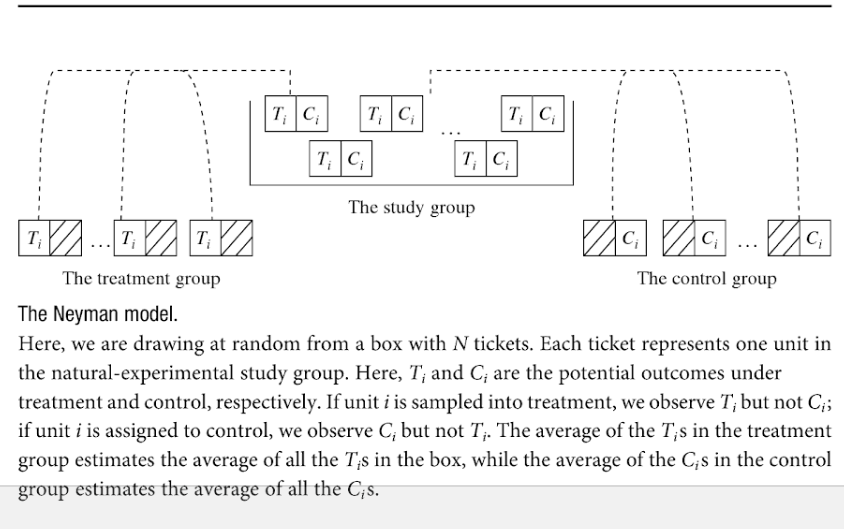March 8, 2019

## Plan for Today:

### (1) Testing Causal Theories

• hypotheses/empirical predictions
• fundamental problem of causal inference

## Hypotheses

#### hypotheses/empirical predictions

statements about what we should observe if the causal claim is true, so stated in terms of independent and dependent variables

• multiple hypotheses based on the overall causal claim and the causal logic
• Hypotheses state relationships between $$X$$ and potential outcomes of $$Y$$
• When $$X$$ is present(absent) for a case, $$Y$$ is present(absent)
• When $$X$$ is present(absent) for a case, $$Y$$ is more(less) likely
• When $$X$$ increases(decreases) for a case, $$Y$$ increases(decreases)

## Potential Outcomes and Hypotheses

Trump's causal claim (implicitly): "The wall caused El Paso to have fewer murders".

• independent variable ($$X$$): presence of wall ($$1$$) or absence of wall ($$0$$) $$Wall_{El \ Paso}$$
• dependent variable ($$Y$$): number of murders $$Murders_{El \ Paso}$$

#### So the hypothesis is…

potential outcomes of $$Murders_{El \ Paso}$$ lower when $$Wall_{El \ Paso} = 1$$

$Murders_{El \ Paso}^{Wall = Yes} < Murders_{El \ Paso}^{Wall = No}$

## Potential Outcomes and Hypotheses

causal claim: "Ownership of firearms by citizens reduces crime"

• independent variable ($$X$$): fraction of citizens owning firearms $$Gun \ Rate_i$$
• dependent variable ($$Y$$): crime victimization rate per 100 thousand $$Crime \ Rate_i$$

#### So the hypothesis is…

Across communities, $$i$$ in $$1 \ldots n$$, on average, potential outcomes of $$Crime \ Rate_i$$ lower for higher values of $$Gun \ Rate_i$$

$Crime \ Rate_i^{Gun \ Rate = High} < Crime \ Rate_{i}^{Gun \ Rate = Low}$

## A big problem

Hypotheses are empirical predictions: they are about what we should observe if $$X$$ causes $$Y$$.

Counterfactual causality means $$X$$ causes $$Y$$ in some specific case, only if potential outcomes of $$Y$$ are different across levels of $$X$$.

But for each case, only one potential outcome becomes "factual" and observable; the other potential outcome(s) remain counterfactual and unobservable

## Fundamental Problem of Causal Inference

We can never observe all of the potential outcomes of $$Y$$ for a case under different exposures to $$X$$. We can only observe the potential outcome of a case that becomes factual where $$X$$ takes on one, specific value. All other potential outcomes of $$Y$$ remain counterfactual and unobservable.

For a single case, we can never empirically observe whether $$X$$ causes $$Y$$

## An Example:

causal claim: "Befriending an immigrant causes people to have positive attitudes of immigrants"

• independent variable ($$X$$): person has immigrant friend $$Friend_i$$: either $$1$$ (yes) or $$0$$ (no)
• dependent variable ($$Y$$): self-reported "overall attitude" towards immigrants $$Attitude_i$$ is $$(-2,-1,0,1,2)$$ for strongly negative, negative, neutral, positive, strongly positive

We collect data on 5 people.

#### Our hypothesis is:

Across persons, $$i$$ in $$1 \ldots 5$$, on average, potential outcomes of $$Attitude_i$$ are higher for higher values of $$Friend_i$$

## An Example:

We collect this data, and we observe this:

$$Person_i$$ $$Friend_i$$ $$Attitude_i$$
1 Yes Positive (1)
2 Yes Very Positive (2)
3 No Neutral (0)
4 No Neutral (0)
5 No Negative (-1)

Can we conclude $$Friend$$ causes more positive $$Attitude$$s toward immigrants?

## An Example:

No, we've forgotten potential outcomes

$$Person_i$$ $$Friend_i$$ $$Attitude_i^{Yes}$$ $$Attitude_i^{No}$$
1 Yes Positive (1) ?
2 Yes Very Positive (2) ?
3 No ? Neutral (0)
4 No ? Neutral (0)
5 No ? Negative (-1)

## An Example:

We want to know the difference in potential outcomes due to $$Friend$$ship: but FPCI means it is unobservable

$$Person_i$$ $$Friend_i$$ $$Attitude_i^{Yes}$$ $$Attitude_i^{No}$$ $$Attitude_i^{Yes} - Attitude_i^{No}$$
1 Yes Positive (1) ? ?
2 Yes Very Positive (2) ? ?
3 No ? Neutral (0) ?
4 No ? Neutral (0) ?
5 No ? Negative (-1) ?

## An Example:

If we were omniscient deities, maybe we could know all potential outcomes: actually, no effect

$$Person_i$$ $$Friend_i$$ $$Attitude_i^{Yes}$$ $$Attitude_i^{No}$$ $$Attitude_i^{Yes} - Attitude_i^{No}$$
1 Yes Positive (1) Positive (1) 0
2 Yes Very Positive (2) Very Positive (2) 0
3 No Neutral (0) Neutral (0) 0
4 No Neutral (0) Neutral (0) 0
5 No Negative (-1) Negative (-1) 0

## Making assumptions:

We make assumptions that let us treat $$Y$$ for cases that we observe with on value of $$X$$ as counterfactuals for cases with different values of $$X$$.

How reasonable/believable are these assumptions?

## One way: Experiments

We cannot know the causal effect of $$X$$ on $$Y$$ for a specific case.

### Experiments (what you NEED to know)

Let us estimate (draw inferences about) the average causal effect of $$X$$ on $$Y$$ for a group of cases.

Three assumptions (you only need to know (1))

1. Random Assignment to "Treatment" and "Control"
2. Exclusion Restriction (only one thing is changing: $$X$$)
3. SUTVA (If I receive the treatment, it doesn't affect you)

## One way: Experiments

Why do experiments work?

### Intuition (you don't NEED to know this)

• In example with immigrant friendship and attitudes about immigrants, people with immigrant friends already different in their potential outcomes (people who hate immigrants don't befriend them)
• Experiments, through random assignment, ensure that potential outcomes of treated/untreated cases are the same (except for random sampling error)

## One way: Experiments

### Social Media Shaming and Voting

Last year's PR referendum

• How many of you voted?

• Would have voted if tagged in a post like this?

"Voting records are public! Records indicate that [name], [name], [name], [name], [name], [name], [name], [name], [name], and [name] have not yet voted in this year’s proportional representation referendum! Voting ends Friday. Click here for voting locations or how to vote from home. [Link]"

## One way: Experiments

### Proof (You REALLY DON'T NEED to know this)